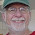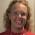Seniors & juniors algebra word problem

Here's a word problem that someone sent me recently:

The total number of girls in the combined junior and senior classes is equal to the number of boys in those two classes. If the senior class has 400 students and the junior class has 300 students, and if the ratio of boys to girls in the senior class is 5:3, what is the ratio of boys to girls in junior class?

This problem gives a lot of information, and it sounds like it can be solved many different ways. But the first task is to notice what we are given and what we are asked.
• We are asked about a ratio
• We're given one ratio, and all kinds of totals. There are boys and girls, senior and junior classes. In other words, there are four groups: senior boys, senior girls, junior boys and junior boys.
This sounds like it can be solved by setting up some equations and using algebra.

To start, you could for example notice the two facts given about the senior class: The senior class has 400 students and the ratio of boys to girls is 5:3.

From this it is easy to solve the number of boys and girls in the senior class.
The ratio 5:3 means 5/8 of them are boys and 3/8 of them are girls. Now, 5/8 of 400 is 250, and 3/8 of it is 150.

So seniors are solved... now to juniors. Let

B1 = boys in junior class
G1 = girls in junior class.

The first sentence of the problem gives us an equation:
G1 + 150 = B1 + 250

We also now that G1 + B1 = 300.

So let's solve this system of two equations:
G1 + 150 = B1 + 250
G1 + B1 = 300

I can subtract the bottom one from the top one to get:

150 - B1 = B1 - 50

200 = 2B1
B1 = 100

Since the total was 300, then G1 is 200.
And now the ratio of boys to girls, it is 100:200 or 1:2. All done!

You could also use straightforward algebra and set up four equations originally, using B1, G1, B2, and G2 for the numbers of boys and girls in junior and senior classes. You would get these four equations:

G1 + G2 = B1 + B2
B2 + G2 = 400
B1 + G1 = 300
B2/G2 = 5/3

And from these four you can solve all four unknowns, and then get the ratio of boys to girls in the junior class.Actually, there are some other useful things to notice: the fact that there are 700 students in total and an equal number of boys and girls tells you that there are 350 of each. So if there are 3/8 of 400 senior girls (i.e., 150), there must be 200 junior girls and 100 junior boys. That's a 1:2 ratio.

That seems like a much more efficient way to get the answer.Maria Miller said…
Yes, I'm not surprised. When I saw this problem, I figured there are many, many ways to solve it. I'm glad you found one of them!

My brain just started doing it a particular way, and I soon got the answer that way. So, that way was "efficient" for me.

I realize sometimes it is worthwhile to spend some time thinking WHICH way would be the most efficient, before even solving the problem, but this was simple enough that that approach is not absolutely necessary.Chi Hung said…
I found your "Seniors & Juniors ..." question pretty interesting. Being a 'model approach' enthusiast, I am able to solve this question without algebra. Please visit http://model4maths.blogspot.com/2011/02/seniors-juniors-algebra-word-problem.html

Appreciate if you can comment on my approach. Cheers.
--Chi HungMaria Miller said…
Chi Hung,
I think the model is great. Like I said, there are many ways to solve this.math software said…
The history of algebra began in ancient Egypt and Babylon, where people learned to solve linear (ax = b) and quadratic (ax2 + bx = c) equations, as well as indeterminate equations such as x2 + y2 = z2, whereby several unknowns are involved. The ancient Babylonians solved arbitrary quadratic equations by essentially the same procedures taught today. They also could solve some indeterminate equations.Heather C said…
When I first read the problem, I was like wow, that's a lot to think about. The way you had solved the problem was my first way as well. Of course there are many ways to set it up and go from there. I think its a great problem for the classroom.How I think about this sort of problem depends on whom I imagine I'm working with. If it's in the context of a classroom math, I look for as many approaches as I can think of, and still imagine I'll get students who see it yet another way.

But when I tutor kids for test prep, efficiency and insight become more important because of time constraints, so I tend to go for what strikes me as clever and quick over pretty much everything.

Here's a problem on a recent ACT:

As shown in the standard (x,y) coordinate plane below, P(6,6) lies on the circle with center (2,3) and radius 5 coordinate units). What are the coordinates of the image of P after the circle is rotated 90 degrees clockwise about the center of the circle?

(The graph is given).

A) (2, 3) B) (3, 2) C) (5, -1)
D) (6, 0) E) (7, 3)

Intriguingly, and to my mind all too predictably, students struggle with this problem because they actually try to do calculations or otherwise ascertain an exact answer. (By the way, it's #37 of 60 problems, so above average in difficulty).

But I would hope that it's obvious to students (operant word being "hope") that a 90 degree rotation about the center of a circle by a point in QI will make the image lie in QIV and hence the coordinates must have signs (+, -) respectively. Thus, C is the only plausible answer. I find many students choose D, which suggests many things: that they aren't thinking about quadrants; that they don't visualize 90 degrees very well in this context; and that in general the propensity to calculate first, rather than think before doing anything is a major source of error on both US standardized tests and, likely, international tests with similar problems used to compare nations.

Doing things the hard way sometimes makes a lot of sense, but here we see that not only is it inefficient considering that students only have 60 minutes for 60 problems of increasing difficulty/trickiness, but also more likely to lead to error.Sam said…
As a reply to what Michael Paul Goldenberg said about the circle problem:

I completely agree that a simple geometric approach is the best bet. As you said, looking at the picture is a lot quicker than solving the geometry, and yields the correct answer.

I disagree, however with what you said "But I would hope that it's obvious to students (operant word being "hope") that a 90 degree rotation about the center of a circle by a point in QI will make the image lie in QIV"

I believe this would only be true the circle was centered at the origin. Since we are rotating the circle off of the origin, its arcs are not equally split up in each quadrant, and the first quadrant contains more than 90 degrees of the circle. (This is evident if you consider the points where the circle meets the positive x and y axis. Clearly the angle from the point on the positive x axis to the center of the circle to the point on the positive y axis is greater than 90 degrees. Thus the point on the circle in the first quadrant just to the right of the y axis, if rotated 90 degrees about (2, 3), would still be in quadrant 1.)Amazingly good one. I thought it could be solved using a lot of methods.